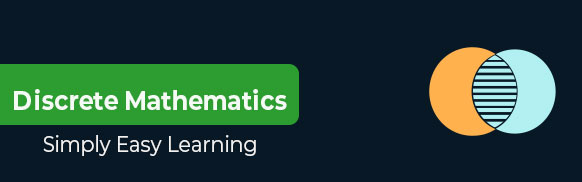# Discrete Mathematics Tutorial

Discrete Mathematics is a branch of mathematics involving discrete elements that uses algebra and arithmetic. It is increasingly being applied in the practical fields of mathematics and computer science. It is a very good tool for improving reasoning and problem-solving capabilities. This tutorial explains the fundamental concepts of Sets, Relations and Functions, Mathematical Logic, Group theory, Counting Theory, Probability, Mathematical Induction and Recurrence Relations, Graph Theory, Trees and Boolean Algebra.

# Audience

This tutorial has been prepared for students pursuing a degree in any field of computer science and mathematics. It endeavors to help students grasp the essential concepts of discrete mathematics.

# Prerequisites

This tutorial has an ample amount of both theory and mathematics. The readers are expected to have a reasonably good understanding of elementary algebra and arithmetic.